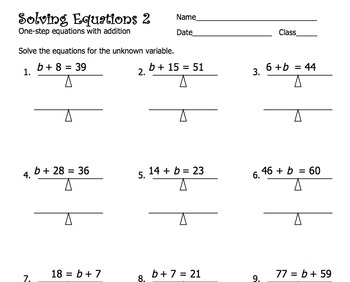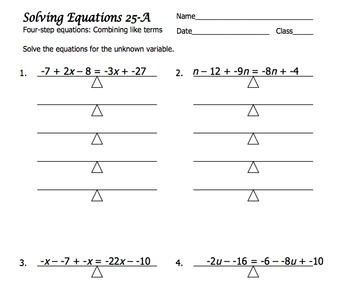# Solving Linear Equations6th - 10th, Homeschool
Subjects
Standards
Resource Type
Formats Included
• PDF
Pages
70 pages

### Description

Over 30 worksheets! If you are searching for a source of linear equations for your students, this is the comprehensive source. Students will begin with one step equations and proceed through subsequent incremental levels until they are solving multi-step problems (involving distribution and combining like terms) with success. Each level begins with positive whole number solutions before introducing negative and fractional solutions. Equations involving addition, subtraction, multiplication, and division are featured sequentially so students can build skills while maintaining comprehension. Each new level is introduced with a visual approach using only positive whole numbers. Then the student is led into more rigorous content involving negative whole numbers and fractions. As students progress, the visual model eventually gives way to more abstract and efficient representations.

Total Pages
70 pages
Included
Teaching Duration
3 Weeks
Report this Resource to TpT
Reported resources will be reviewed by our team. Report this resource to let us know if this resource violates TpT’s content guidelines.

### Standards

to see state-specific standards (only available in the US).
Apply the properties of operations to generate equivalent expressions. For example, apply the distributive property to the expression 3 (2 + 𝘹) to produce the equivalent expression 6 + 3𝘹; apply the distributive property to the expression 24𝘹 + 18𝘺 to produce the equivalent expression 6 (4𝘹 + 3𝘺); apply properties of operations to 𝘺 + 𝘺 + 𝘺 to produce the equivalent expression 3𝘺.
Solve real-world and mathematical problems by writing and solving equations of the form 𝘹 + 𝘱 = 𝘲 and 𝘱𝘹 = 𝘲 for cases in which 𝘱, 𝘲 and 𝘹 are all nonnegative rational numbers.
Solve multi-step real-life and mathematical problems posed with positive and negative rational numbers in any form (whole numbers, fractions, and decimals), using tools strategically. Apply properties of operations to calculate with numbers in any form; convert between forms as appropriate; and assess the reasonableness of answers using mental computation and estimation strategies. For example: If a woman making \$25 an hour gets a 10% raise, she will make an additional 1/10 of her salary an hour, or \$2.50, for a new salary of \$27.50. If you want to place a towel bar 9 3/4 inches long in the center of a door that is 27 1/2 inches wide, you will need to place the bar about 9 inches from each edge; this estimate can be used as a check on the exact computation.
Use variables to represent quantities in a real-world or mathematical problem, and construct simple equations and inequalities to solve problems by reasoning about the quantities.
Solve word problems leading to equations of the form 𝘱𝘹 + 𝘲 = 𝘳 and 𝘱(𝘹 + 𝘲) = 𝘳, where 𝘱, 𝘲, and 𝘳 are specific rational numbers. Solve equations of these forms fluently. Compare an algebraic solution to an arithmetic solution, identifying the sequence of the operations used in each approach. For example, the perimeter of a rectangle is 54 cm. Its length is 6 cm. What is its width?